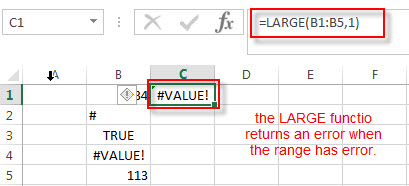# Excel LARGE Function

This post will guide you how to use Excel LARGE function with syntax and examples in Microsoft excel.

## Description

The Excel LARGE function returns the largest numeric value from the numbers that you provided. Or returns the largest value in the array. It also can be used to get the nth largest value from a range of cells or an array.

For example, the LARGE function can find the first, second, third or nth smallest value in a list or an array.

The LARGE function is a build-in function in Microsoft Excel and it is categorized as a Statistical Function.

The LARGE function is available in Excel 2016, excel 2013, excel 2010, excel 2007, excel 2003, excel XP, excel 2000, excel 2011 for Mac.

## Syntax

The syntax of the LARGE function is as below:

`= LARGE (array,nth)`

Where the LARGE function arguments are:

• array -This is a required argument.  An Array or a range of cells that contains numeric values from which you want to get the nth largest value.
• nth – This is a required argument.  The position of the number that you want to return

Note:

• If the first argument is empty, then the LARGE function returns #NUM! Error.
• If nth is less than or equal to 0 or if nth is greater than the number of values in array, the LARGE function returns the #NUM! Error.
• If nth is the number of values in array, then LARGE (array,1) returns the largest value, and LARGE(array,n) returns the smallest value.

## Excel LARGE Function Examples

The below examples will show you how to use Excel LARGE Function to get the largest value form the numbers in a range.

#1 To get the largest value from a range B1:B4, just using the following excel formula:

`=LARGE(B1:B4,1)`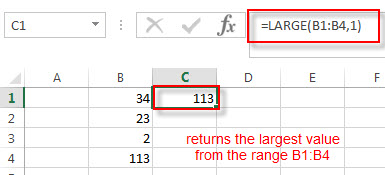The above formula returns the largest number from a range B1:B4, it returns 113.

2# To get the second largest value from the Range B1:B4, you can use the following LARGE function:

`=LARGE(B1:B4,2)`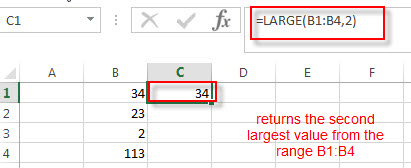The second argument nth in the above LARGE function is 2, so it will fetch the second largest function in the range B1:B4. It returns 34.

3# To get the largest value from the range B1:B4 that contains blank cells, enter into the following formula in the Cell C1:

`=LARGE(B1:B4,1)`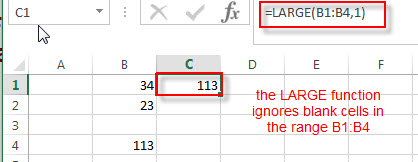The LARGE function will ignore blank cells in the Range. You will see that the Cell B3 is a blank cell, and it is ignored by the LARGE function. The largest value is still returned as 113.

4# To get the largest value from the range B1:B5 that contains text string, specific character and logical values. Using the following LARGE formula:

`=LARGE(B1:B5,1)`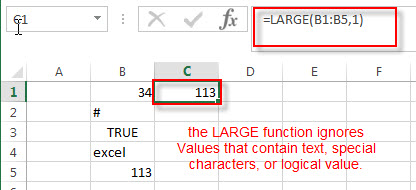The LARGE function will ignore values in the range B1:B5 that contain text string, specific character and logical value.

5# To get the largest value from the range B1:B5 that contain an error, using the below formula:

`=LARGE(B1:B5,1)`### More Excel LARGE Function Examples

• Find the Largest Value Based on Multiple Criteria
Assuming that you have a list of data that you want to find the largest value based on the product “excel” and the sales region “east”. You can create a new excel formula based on the SUMPRODUCT function and the LARGE function..……
• Find Closest Value or Nearest Value in a Range
You need to use an excel array formula based on the INDEX function, the MATCH function, the MIN function and the ABS function to find Closest Value or Nearest Value in a Range in Excel…